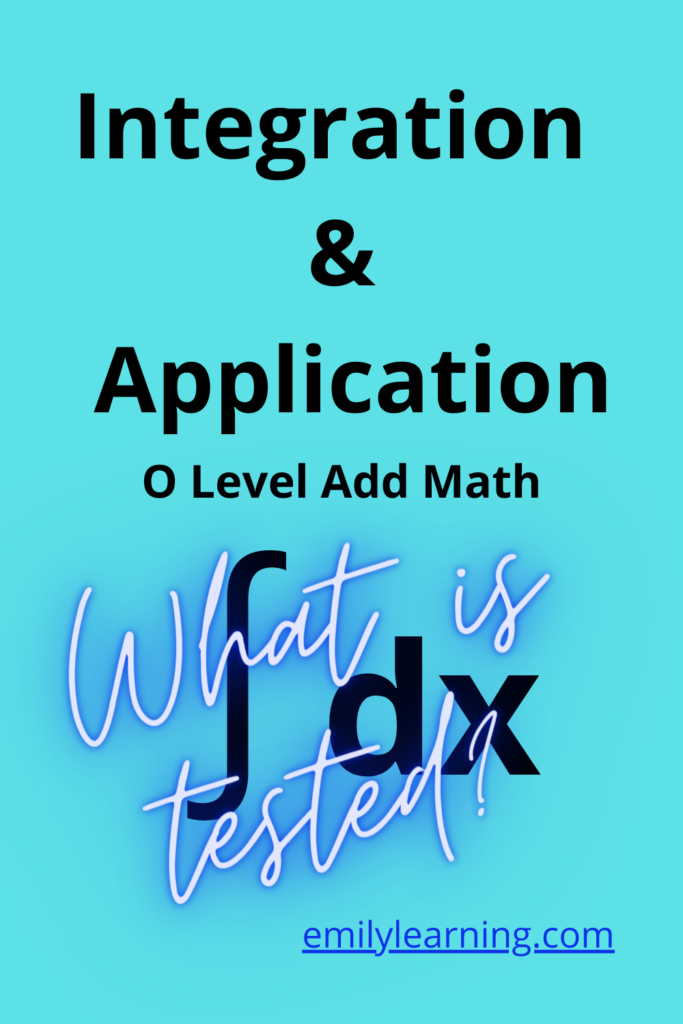# What you need to know for integration and its application as tested in O Level Additional MathematicsIntegration is a big topic in O Level Additional Mathematics, just like differentiation. Integration and differentiation make up what often call calculus. Just like differentiation, you are also required to know concepts from previous topics such as trigonometry, exponentials, and logarithms as you are required to do some manipulation of trigonometric, exponential, and logarithmic functions occasionally.

There are only so many functions that you can integrate directly. Hence, there are lesser formulae for integration. Also, there’s no product rule or quotient rule for integration, so there is less content on integration tested in O level compared to differentiation. But, because integration and differentiation often go hand-in-hand (since integration is the reverse of differentiation), there are many questions at O level that involves both differentiation and integration within the same question. I go through integration, its application in detail in my course on integration and its application. This course also includes the application of integration and differentiation to kinematics which I talk more about here. You can get my online on-demand course on Integration, Application of Integration, Kinematics (Displacement, Velocity, and Acceleration) here. These are what you need to know for integration and its application. Make sure you know all of them prior to your O Level Add Maths exam!

These are what you need to know for integration and its application. Make sure you know all of them prior to your O Level Add Maths exam!

• integration of
• algebraic expressions axn and (ax+b)n
• trigonometric functions sin(ax+b), cos(ax+b) and sec2(ax+b)
• integration of 1/ (ax+b)
• integration of e(ax+b)
• finding definite integrals
• finding area using integration
• application of integration as the reverse of differentiation

We go through integration and its application in detail in our course. In addition, we also include the entire chapter on the application of integration and differentiation to kinematics (displacement, velocity, and acceleration) in this course. Get our on-demand course here to start learning these topics well now.

error: Content is protected !!